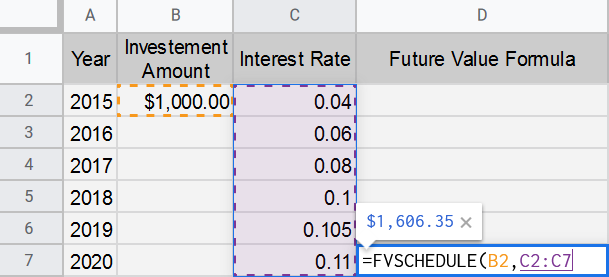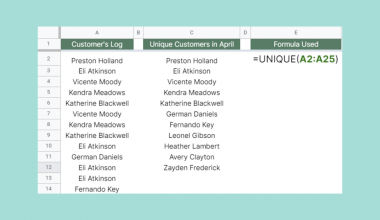# How to Use FVSCHEDULE Function in Google Sheets

The FVSCHEDULE function in Google Sheets is useful when you want to know the future value of an investment at variable compound interest rates. Interest rates can be different in some or all of the years of the investment tenure.

This  function calculates the future value of a number, after some years of being multiplied annually by compound interest values. If you haven’t figured it out, FV stands for “Future Value”.m

So, what’s compound interest? Let’s see an example to illustrate the idea.

Let’s say you have an original amount of \$1000 in your bank account, and your bank contract states that there’s an annual 10% compound interest. This means that next year you will have 1.1 multiplied by your original amount, which equals \$1100. Now in year two, you would have 1.1 multiplied by your previous year’s amount, not your original value, so \$1100 * 1.1 which equals \$1210. That’s what compound interest means.

Now, if we apply the same example to simple interest, your value would be \$1300 after two years instead of the \$1210 of the compound interest.

In other words, simple interest is based on the original amount of a loan or deposit, and compound interest is based on the original amount and the periodical added interest.

The `FVSCHEDULE` function can take several amounts of interest rates, where their count  represents the number of periods upon which the original value has increased. Let’s see how that goes in the following section.

## The Anatomy of the FVSCHEDULE Function in Google Sheets

So, the syntax (the way we write) of the `FVSCHEDULE` function is as follows:

`=FVSCHEDULE(principal, rate schedule)`

Let’s dissect this thing and understand what each of these terms means:

• = (the equal sign) is just how we start any function in Google Sheets.
• `FVSCHEDULE` is the name of the function we are using.
• () These parentheses are used to host the two values we put in our function, and a comma “,” must separate these values.

Note that the values hosted in any google sheets function are called arguments.

• (principal) The function’s first argument is the argument that takes the original value of our investment, loan, or deposit.
• (rate schedule) The function’s second argument takes the values of the interest rates; however, they may vary.

Also, note that if any of these two arguments weren’t specified, the function would result in an error.

Now for further explanation, let’s go through the `FVSCHEDULE` function together, and you will understand it once you start practicing its application.

## A Real Example of Using FVSCHEDULE Function

Take a look at the example below to see how the `FVSCHEDULE` function is used in Google Sheets.As you can see in the table, the Future Value Formula column has the formula of the `FVSCHEDULE` function, and the Future Value Result column has the formula’s result.

Now let’s look closely at the formula we have.

=`FVSCHEDULE` (B2, C2:C7)

It can also be written as follows.

=`FVSCHEDULE` (1000, {0.04, 0.06, 0.08, 0.1, 0.105, 0.11})

Where in the second version, the range of interest rates has been written manually in the formula as a list.

So, what does this function do?

Here’s the equation being calculated in the background.

Value = \$1000 * 1.04 * 1.06 * 1.08 * 1.1 * 1.105 *1.11 = \$1606.35

And if we add one year with an interest rate of 0.115 the value would be \$1606.35 * 1.115 which is equal to \$1791.

Now that we perfectly understand the concept of the `FVSCHEDULE` function, let’s begin writing our `FVSCHEDULE` function in Google Sheets.

You may make a copy of the spreadsheet using the link I have attached below.

## How to Use FVSCHEDULE Function in Google Sheets

1. Simply click on any cell to make it the active cell. For this guide, I will be selecting D7, where I want to show my formula. Then type “=”.
2. Now, type “FVS” and click on the `FVSCHEDULE` function or press Tab to select it.3. Now, select the principal (original) value of our investment which is the value in cell B2. Here you can write “B2” or “1000“.4. Then, type comma “,”.
5. Now select the range of interest rates (C3:C7), which as mentioned before, you can also type down manually in a list as follows: “{0.04, 0.06, 0.08, 0.1, 0.105, 0.11}“.6. Finally, close the parentheses and press Enter, and your result will take over.

Finally, know that you can dissect the formula into six different formulas to get the same result, but there’s a catch. Check out the following illustration to understand.Notice that in the first formula, the one in D2, we used the original investment amount (B2) in the first argument. While in each of the next formulas, we use the value of the result in the previous formula as a first argument.

If we were dealing with simple interest, the first argument would have been the same for all six formulas.

That’s pretty much it. Congrats! You now mastered the `FVSCHEDULE` function in Google Sheets.

There are many more useful Google Sheets functions that you can enjoy learning daily.##### You May Also Like## How to Create Dot Plots in Google Sheets

Learning how to create dot plots in Google Sheets is useful for creating a simple and easy-to-understand representation…## How to Use RANK Function in Google Sheets

The RANK function in Google Sheets is used to determine the rank of a specific value in a…## How to Use UNIQUE Function in Google Sheets

The UNIQUE function in Google Sheets is useful if you want to extract unique values from a given…## How to Use DAVERAGE Formula in Google Sheets

The DAVERAGE formula in Google Sheets is useful to get the average of values that meet specific criteria.…